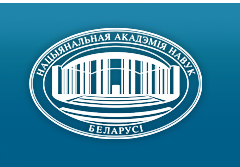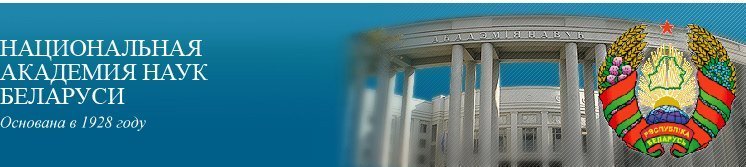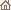Главная Новости Об академии Деятельность академии Члены академии Организации Издания академии Мероприятия Контакты Электронные обращения Справочная информация Карта сайта

Известия Академии наук Беларуси. Сер. физ.-матем. наук, 1997, No.4/ Издания академии / Научные журналы

Известия Академии наук Беларуси. Сер. физ.-матем. наук, 1997, No.4Известия Академии наук Беларуси СЕРИЯ ФИЗИКО-МАТЕМАТИЧЕСКИХ НАУКИздатель: Беларуская навука, Минск, Республика Беларусь

Номер 4, 1997СОДЕРЖАНИЕ

МАТЕМАТИКА

Yegorov A.D.
On functional quadrature formulae for integrals with respect to the measures generated by solutions of stochastic differential equations. pp. 5--8

Summary: Wiener functionals based approach to approximate evaluation of continual integrals with respect to the measure generated by solution of Ito's stochastic equation is developed.

Harin Yu.S., Stalevskaya S.N.
On stability of multivariative linear regression forecasting. pp. 9--13

Summary: Problem of statistical forecasting for the observation model defined by multivariate linear regression in presence of Tukey -- Huber "outliers" and random distortions of predictors is considered. Asymptotic expressions for the risk of forecasting, for the bias of forecast and for the factor of relative risk increment are given and investigated.

Laptinski B. N.
On periodic solutions of nonlinear matrix differential equations. pp. 14--18

Summary: Iterative methods are proposed to construct periodic solutions of nonlinear matrix differential equations.

Yudin M.D.
On the limit distributions of dependent vector sums. pp. 19-23

Summary: Based on generalization of the Levy -- Khinchin formula, the central limit theorem of probability theory is solved for dependent random vector sum.

Blinova Ye.I., Zalesski B.A.
Hierarchical algorithm for recovering of bilevel images. pp. 24--29

Summary: The paper is devoted to recovering of bilevel images sampled with random noise. The bilevel images are interpreted as functions on the unit square, which can take only two values. The hierarchical algorithm consist in detecting parts of the square in which the function is constant, from large parts to small ones. The Ls-risks of recovering are estimated in terms of Besov space norms. It is shown that this estimates can not be improved up to logarithmic factor. The result for d-dimensional case is formulated, too.

Approximate evaluation of Wiener's continual integrals in some special cases. pp. 30--34

Summary: Some numerical methods for evaluation of continual integrals on Wiener's measure on the space of continuous functions from functionals f(ò 01 p2(t) x2(t) dt ). Our methods have the complexity O(n) for the exponential case and O (n2 N2) for the general one, where n is the multiplicity of integral that approximates the continual one, N is the capacity of grid for numerical evaluations on the line.

Ignatenko M.V., Yanovich L.A.
Approximate evaluation of continual integrals with respect to gaussian measures. pp. 35--41

Summary: The Lagrange interpolation formulas with respect to knots of the second multiplicity for differentiable and nondifferentiable operators defined on the sets of functions are constructed.

Leonov N.N.
The structure of metrics and isometric embeddings into ultrametric spaces. pp. 42--45

Summary: Some theorems concerning the range of the metric (distance) in an ultrametric space are proved. These theorems are used to investigate isometric embeddings of ultrametric spaces.

Degtyarenko N.A.
Solution in closed form of a convolution type integral equation with four kernels and conjugation. pp. 46--51

Summary: An integral equation of the convolution type with four kernels and conjugation is considered. It is reduced to the Riemann problem which can not be solved by the traditional methods. New restrictions on the coefficients of the Riemann problem, which provides its solvability, are found. The techniques of solving the Riemann problem of Riemann surfaces is actually used. Solution of the equation in its closed form is given.

Baranov A.A.
On Lie subalgebras of locally finite-dimensional associative algebras. pp. 52--56

Summary: Locally finite-dimensional Lie algebras that can be embedded into locally finite-dimensional associative algebras are studied. Some results about simple ones are announced.

Mirotin A.P.
On functional calculus of dissipative elements of Banach's algebras. pp. 57--61

Summary: We show that under certain conditions the norm and the spectral radius for an absolutely monotone function of a dissipative element of a Banach algebra coincide.

Selkin M.V., Sidorov A.V.
On intersections of maximal subgroups of finite groups. pp. 62--64

Summary: The properties of intersections of maximal subgroups of a finite group G containing Op(G) for a certain set of primes p are considered.

Aniskov V.V.
On reducible local formations of a given N-defect. pp. 65--68

Summary: A local formation F is called reducible if F = Iform (Èi Î I ) Fi, where {Fi : i Î I} -- is the set of all nontrivial local subformations of F. We obtain the exact description of reducible local formations of finite groups which have h-defect 1 and 2 for any 2-multiply local formation h.

Avdashkova L.P.
A question about the subnormal closure. pp. 69--72

Summary: For soluble S-closed local formation F, the F-subnormal closure of a subgroup of an arbitrary finite group is described.

Sobolevskaya E.P.
Knapsack problem with polymatroidal constraints. pp. 73-76

Summary: The problem of integer linear programming on the base-polyhedron of a submodular function under an additional linear constraint is considered. For an approximate solution of the problem, algorithm of Lagrange's function minimization is proposed.

ФИЗИКА

Bogush A.A.
On four-dimensional quantum vector parameters of q-deformed groups. pp. 77--81

Summary: A description of q-deformed groups GLq(2.C), SLq(2) in terms of four-dimensional quantum vector parameters is given. A simple composition rules and basic commutation relations for these q-deformed vector-parameters are introduced.

Borodich A.I.
Calculation of the inverse operator of phase coordinates transformation for particles of the Hamiltonian system. pp. 82--86

Summary: Explicit equations for operator polynomials used to factorize the inverse transfer map in order to calculate the phase space coordinates of any particle of the Hamiltonian system whose nonlinear behavior description is related to given trajectory parameters are obtained.

Tuniak U.M.
Weak conservation law and non-coordinate gauge transformations for scalar-tensor theory of gravitation in the Minkowski space. pp. 87--90

Summary: In the flat Minkowski space, we develop gravitational theory of scalar-tensor field with massive tensor component. A relation for the total conserved energy-momentum tensor of the matter and of gravitational field in the Minkowski space is obtained. The concept of non-coordinate gauge transformations is considered.

Barvenov S.A.
Influence of variable cinematic viscosity coefficient on wave motions of fluid caused by periodic surface disturbance. pp. 91--95

Summary: Forced periodic motion of viscous fluid caused by changing pressure is considered. It is obtained that the coefficient of cinematic viscosity can be an arbitrary function of function of the depth.

Karolik A.S., Golub V.M.
Application of the augmented plane-wave method to calculation of the electronic structure and heat capacity of Cu-Ni alloys in the virtual crystal approximation. pp. 96--101

Summary: The electronic band structure and low-temperature specific-heat coefficient of substitutional disordered paramagnetic Cu--Ni alloys are calculated using the virtual crystal approximation on the base of augmented plane-wave method. The non-self-consistent MT-potential is built using the Hartree -- Fock -- Roothan atomic wave function with a-exchange correction, satisfying the virial theorem. The results of calculation are in a quite satisfactory accordance with the experimental data.

Sirota N.N., Beresina G.M., Vitkina C.S., Yanowich V.D.
Influence of mechanical treatment and radiation on structural properties of cellulose. pp. 102--104

Summary: The crystallinity degree, grain size, and deformations of the crystal lattice in the microcrystalline and fibrous cellulose appearing after crushing and radiation are determined by X-ray diffraction method.

Tochitski T.A.
On the twinning mechanism in electrodeposited magnetic films with FCC lattice. pp. 105--108

Summary: Analysis of the twinning process in the electrodeposited magnetic films having a FCC lattice is done.

Nuprienok I.S., Cheplanov A.M., Shibko A.M.
Influence of combined processing on electrophysical properties of titanium-silicon composition contact. pp. 109--111

Summary: Peculiarities of phase composition and electrophysical properties of titanium-silicon composition under combined processing are studied. Light irradiation of heated materials stimulates redox reaction power and the material temperature. Light thermal treatment of the Ti--Si composition makes it possible to form a rectifying contact with given parameters depending on light irradiation power.

Makovetski G.I., Smolik Ch.K., Severin G.M.
Linear smoothing errors of physical experiment based on extrapolation. pp. 112--116

Summary: New method for smoothing experimental data based on the even approximation with the help of the discrete linear transformation of convolution. The proposed method exceeds the spline methods by accuracy and increases resistance to measurement errors.

ИНФОРМАТИКА

Yemelichev V.A., Yefimchik N.Ye.
On effective algorithm, which solves the problems of allocation of medians and centers in graphs in typical cases. pp. 117--120

Summary: We propose a statistically efficient algorithm for solving simultaneously both problems of location of k medians and k centers on a complete weighted directed graph. It is proved that the proposed approximate algorithm gives exact solutions of both problem "almost always" (in most cases) under certain restrictions on the cardinality of the set of weights.

КРАТКИЕ СООБЩЕНИЯ

Sirota N.N.
On thermodynamic stability of amorphous phase. pp. 121--122

Summary: Conditions of stability of an amorphous condensed phase in the certain temperature range and at low temperatures up to the absolute zero are considered.

Vasilevich N.D., Prohorovich T.N.
On a special case of the Fuch system. pp. 123--125

Summary: The solution of Fuch's system with a special monodromy group is obtained.

Benediktovich I.I.
A remark on the degree of the standard associative identity for the classical Lie algebras. pp. 126--127

Summary: The degree of the minimal standard associative identity for some classical Lie algebras with respect to standard matrix representation is calculated.

АННОТАЦИИ ДЕПОНИРОВАННЫХ СТАТЕЙ

Monastyrny P.I., Kremen' Ye.V., Kremen' Yu.A.
Optimization of Godunov's method and computational experiment for solving three point difference equation. p. 128

Kremen' Ye.V.
On the method of multiple difference trial shooting for systems of nonlinear multipoint difference equations with nonseparated boundary conditions. pp. 128--129

Mateiko O.M.
On some two-generated subgroups of group PSL2(C). p. 129

Hapaliuk A.P.
On certain singularities of the generalized Fourier transform. p. 130

Savchuk V.F., Matysik O.V.
Iteration stop rule for the iterative method of solving linear equations. p. 130

УЧЕНЫЕ БЕЛАРУСИ

Аляхновіч М.М., Апанасевіч П.А., Архіпенка В.І. і інш.
Аляксандр Паўлавіч Вайтовіч (Да 60-годдзя з дня нараджэння). C. 131--133
Нажмите
здесь для чтения статьи

Вайтовіч А.П., Лесніковіч А.І., Ільін В.М., Аляхновіч М.М.
Анатоль Паўлавіч Дастанка (Да 60-годдзя з дня нараджэння). C. 134--135
Нажмите здесь для чтения статьи

Скіба А.М., Манахаў В.С., Каморнікаў С.Ф., Селькін М.В.
Леанід Аляксандравіч Шамяткоў (Да 60-годдзя з дня нараджэння). C. 136--137
Нажмите здесь для чтения статьи

ХРОНИКА

Gordon V.S.
In the Belarusian Society for Operation Research. p. 138/ Издания академии / Научные журналы / К началу страницы

Разработана и поддерживается Николаем Н. Костюковичем. Последнее обновление: 7 ноября 2006 г.
Создана при участии Игнатия И. Корсака
Копирайт © 1998-2006 Национальная академии наук Беларуси
Копирайт © 1997 Издательство "Беларуская навука"